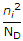# Electronics and Communication Engineering - Electronic Devices and Circuits

31.

Assertion (A): In n-p-n transistor conduction is mainly due to electrons.

Reason (R): In n type materials electrons are majority carriers.

 A. Both A and R are true and R is correct explanation of A B. Both A and R are true but R is not a correct explanation of A C. A is true but R is false D. A is false but R is true

Answer: Option A

Explanation:

No answer description available for this question. Let us discuss.

32.

The factor n in the equation for calculating current for a silicon diode is

 A. 1 B. 2 C. 2.5 D. 2 for low levels of current and 1 for high levels of current

Answer: Option D

Explanation:

No answer description available for this question. Let us discuss.

33.

An LED is

 A. an ohmic device B. a display device C. a voltage regulated device D. all of the above

Answer: Option B

Explanation:

No answer description available for this question. Let us discuss.

34.

An enhancement mode MOSFET is on when the gate voltage is

 A. zero B. positive C. high D. more threshold value

Answer: Option D

Explanation:

No answer description available for this question. Let us discuss.

35.

For the n-type semiconductor with n = NP and P =, the hole concentration will fall below the intrinsic value because some of the holes

 A. drop back to acceptor impurity states. B. drop to donor impurity states C. virtually leave the crystal D. recombine with the electrons.

Answer: Option D

Explanation:

No answer description available for this question. Let us discuss.

#### Current Affairs 2021

Interview Questions and Answers